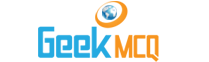Engineering :: Mechanical Measurements

1.  If a steam pressure of 10 kg/cm2 is to be measured, the range of pressure gauge should be
 A. ?10 kg/cm2 to + 10 kg kg/cm2 B. 0 to 10 kg kg/cm2 C. 5 to 11 kg kg/cm2 D. 0 to 20 kg kg/cm2 E. 0 to 100 kg kg/cm2

2.  The degree of perfection used in instruments, the methods and the observations, is known as
 A. Precision B. Accuracy C. Efficiency D. Least count E. Error

3.  The accuracy depends upon
 A. Precision of instrument B. Precision of method C. Good planning D. All of the above E. None of the above

4.  A discrepancy is
 A. The difference between a measurement and true value of the quantity measured B. The difference between true value of the quantity and error C. The difference between the measured value and actual value D. The difference between the measured values of the same quantity E. None of the above

5.  If a measuring tape is too long as compared to standard, the error will be known as
 A. Instrumental error B. Personal error C. Natural error D. Manufacturing error E. Superficial error

6.  Natural error in measurement may be due to
 A. Humidity B. Temperature C. Wind D. Gravity E. Any of the above

7.  The errors which form inexperience of the observer are known as
 A. Training errors B. Handling errors C. Personal errors D. Accidental errors E. Mistakes

8.  If an error under the same conditions will always be of the same size and sign, it is known as
 A. Training errors B. Systematic errors C. Cumulative error D. Either of (A) and (B) above E. Either of (B) and (C) above

9.  The statement "The most probable value of an observed quantity available from a given set of observation is the one for which the sum of the square of errors is a minimum" is known as
 A. Law of square probability B. Pythogorus theorem C. Principle of least squares D. Law of errors E. Principle of square errors

10.  The maximum allowable limit that a measurement may vary from the true value is called
 A. Permissible error B. Expected error C. Range of error D. Least error E. Safe error